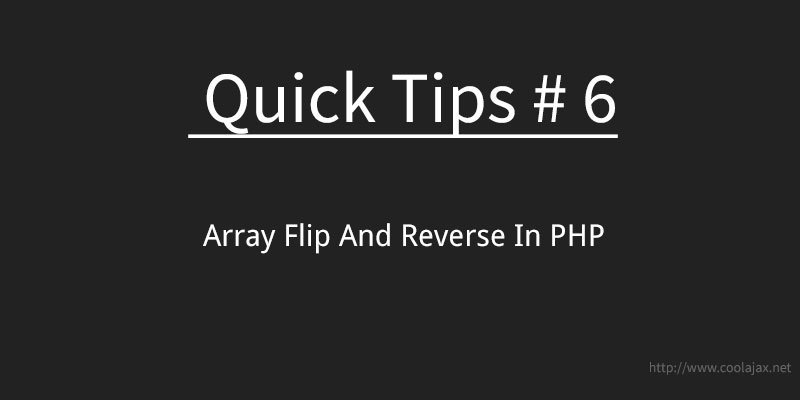# Array flip and reverse in phparray_flip function is quite useful when you only want to shift array index as array value and vice-verse. On the other hand, if you want to change only array index in descending/reverse order then array_reverse function works great.

Array flip convert key in to index and index in to key.

```\$a = array (0 => "a", 1 => "b", 2=>"c");
print_r (array_flip (\$a));

// Output:
// Array ( [a] => 0 [b] => 1 [c] => 2 )```

Array reverse function changes the order of array value.

```\$a = array (0 => "a", 1 => "b", 2=>"c");
print_r (array_reverse (\$a));
// Output
// Array (  => c  => b  => a )```